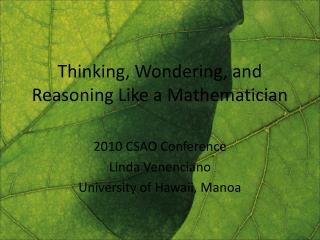DownloadDownload PresentationThinking, Wondering, and Reasoning Like a Mathematician

# Thinking, Wondering, and Reasoning Like a Mathematician

Télécharger la présentation## Thinking, Wondering, and Reasoning Like a Mathematician

- - - - - - - - - - - - - - - - - - - - - - - - - - - E N D - - - - - - - - - - - - - - - - - - - - - - - - - - -
##### Presentation Transcript

1. Thinking, Wondering, and Reasoning Like a Mathematician 2010 CSAO Conference Linda Venenciano University of Hawaii, Manoa

2. Example 1 Solve: 342 -145 How would a student be taught to do this? What thinking process is demanded of the student? Of the teacher? Is this a problem or an exercise?

3. Example 2 • Using the digits 1 to 9 only once, find two 3-digit numbers whose sum uses the remaining three digits. How would a student be taught to do this? What thinking process is demanded of the student? Of the teacher? Is this a problem or an exercise?

4. Thinking Like a Mathematician • What does a mathematician do? • Investigate, solve problems, reason, prove, justify, communicate, validate through collaboration • Is their objective to get “the right answer”? • Some problems take a very, very, very long time to solve • It’s the means • Applied mathematics vs. pure mathematics

5. Thinking, Wondering, Reasoning Describe the nature of school mathematics. How do children learn to think mathematically and problem solve?

6. Problem Solving Tips • Patience • Try something • Draw a picture • Organize your findings • Look for patterns • Generalize • Look at simpler problems first • Wish • What if x=1? What if x=10?

7. Characteristics of a good problem Skeleton tower example • a. How many cubes are needed to build this tower? Explain how you found the total. • b. How many cubes are needed to build a similar tower twelve cubes high? Explain how you found the total.

8. ULS HS Textbook Selection Criteria • Mathematics meet standards and benchmarks • Learning through problem solving is included as an instructional strategy • Topics are well connected • Multiple means of communication are present • A variety of instructional strategies are used • Multi-dimensional assessment techniques are available • Professional development support is available • Modern instructional technology is integrated • Appropriate balance between concepts and skills • Well developed formative and summative assessment • Multiple representations are addressed and the connections between the representations are made explicit

9. Instruction • Interactions to build understanding • Promote productive problem solving processes • Prompt students to go beyond finding the right answer • Broaden student participation • Expand student engagement

10. Resources • SUPER-M project • http://www2.math.hawaii.edu/superm/ • NSF Graduate STEM Fellows in K-12 Education • http://www.gk12.org/ • Math Teachers’ Circle Network • http://www.mathteacherscircle.org/ • Curriculum Research & Development Group • http://www.hawaii.edu/crdg/pd/

11. GK-12 is an NSF-funded Program • Program Goals for Graduate students, K-12 Education, and Higher Education • Fellows have the unique opportunity to improve their communication and teaching skills through interactions with teachers and students in K-12 schools

12. GK-12 Outreach Work • Producing an event, “Molokai Math Day” • Working with the community to develop their infrastructure and enjoy a day of mathematics activities with families on Molokai • Designing and delivering two CRDG Summer Programs courses for 2010 • International Week of Math • PD through HCTM, our own workshops, our school partners